﻿ Optimization of Semi-active Seat Suspension

### Optimization of Semi-active Seat Suspension

Stefan Segla, N. TrišovićOPEN ACCESSPEER-REVIEWED

## Optimization of Semi-active Seat Suspension

Stefan Segla1,, N. Trišović2

1Department of Mechanical Engineering, Technical University of Košice, Košice, Slovakia

### Abstract

This paper deals with modeling and optimization of the dynamic characteristics of a working machine semi-active suspension seat. The seat suspension is composed of a spring parallelly ordered with a semi-active damper controlled by the sky-hook control algorithm. The paper also points on the possibility of improving the dynamic characteristics of the seat with the use of a dynamic vibration absorber. The dynamic characteristics of the suspensions were optimized using a two-objective function, where besides the component respecting the effect of the effective acceleration of the seat also the effect of the effective relative displacement between the seat and the floor of the working machine cabin was considered. The first component expresses comfort of the seat and the second one expresses the safety of machine handling. The semi-active seat suspension provides significant improvement of the seat dynamic characteristics compared with passive suspensions. Further improvement was brought by the dynamic vibration absorber.

### At a glance: Figures

123
Prev Next

• Segla, Stefan, and N. Trišović. "Optimization of Semi-active Seat Suspension." American Journal of Mechanical Engineering 1.7 (2013): 221-225.
• Segla, S. , & Trišović, N. (2013). Optimization of Semi-active Seat Suspension. American Journal of Mechanical Engineering, 1(7), 221-225.
• Segla, Stefan, and N. Trišović. "Optimization of Semi-active Seat Suspension." American Journal of Mechanical Engineering 1, no. 7 (2013): 221-225.

 Import into BibTeX Import into EndNote Import into RefMan Import into RefWorks

### 1. Introduction

The primary objective of the working machine seat suspension is to ensure damping of mechanical vibration to achieve the desired comfort of the working machine driver (operator) and also the safety of the machine handling. These requirements act in a certain way in opposite, therefore multi-objective optimization is necessary [1, 2, 3, 4, 5, 6]. Usually comfort of the driver is characterized by the seat acceleration and the safety of the machine handling (driver’s clear sightedness) by the relative displacement between the seat squab and the working machine cabin floor.

Nowadays three types of seat suspension are used. The most used are still passive suspension systems [7, 8] with conventional springs (often pneumatic) and hydraulic dampers. Pneumatic springs and hydraulic dampers today allow to adjust the coefficient of stiffness of the spring and also the damping coefficient of the damper, which enable individual settings of the seat suspension parameters according to the subjective perception of the working machine driver.

The passive suspensions however reached their top and therefore active suspension systems [9, 10] are gradually more and more used in practice. Their dynamic characteristics are significantly better compared to passive suspension systems, but they are still more expensive, more complex and therefore they are less reliable. They also require an external source of energy and their energetic demand is high.

A compromise between passive and active suspension systems are semi-active suspension systems [11, 12, 13], which can be realized by the use of magnetorheological (nowadays the most used), electrorheological or friction dampers.

Application of dynamic vibration absorbers enable further improvement of the dynamic characteristics of suspension systems [14, 15]. The application of the dynamic vibration absorber is possible only in applica-tions where the frequency characteristics of the excitation do not change significantly.

In the paper the dynamic characteristics of the semi-active suspension of working machine seats will be optimized, whereby the results of the optimization with or without a dynamic absorber will be compared.

### 2. Dynamic and Mathematical Models of the Semiactive Suspension

As the dynamic and mathematical models of the semi-active suspension differentiate with or without the use of the dynamic vibration absorber (not the principle of their modeling and optimization), there will be shown both cases in the following parts of the paper.

2.1. Dynamic and Mathematical Models of the Semi-active Suspension without the Dynamic Vibration Absorber

The dynamic model of the seat, shown in the Figure 1, is composed of the mass of the seat squab and parts of the seat connected with it and machine operator (ms+mop), spring with the equivalent coefficient of stiffness k (which can be realized for example by an air bag, what enables easy adjustments in the elevation and its coefficient of stiffness) and a collaterally ordered semi-active damper with a continuously varying coefficient of damping csa.

The equation of motion of the semi-active suspension without the dynamic vibration absorber according to the Figure 1 can be written in the form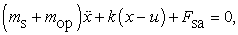(1)

where x is the coordinate of the seat squab mass ms+mop. Fsa is the damping force generated by the semi-active damper and u(t) represents the kinematic excitation of the seat. Its time dependence was obtained experimentally in the driver’s cabin (under the driver’s seat in the vertical direction) in the bucket wheel excavator Schrs 1320 on a coal land mine Bílina (Czech Republic). The time dependence of the excitation displacement u(t) is shown in the Figure 2.

The sky-hook control algorithm will be considered. The task of the damper is to generate the same force, with the same direction and magnitude as the fictitious damper on the seat would generate, the so called sky-hook damper placed between the seat and a point, which location in the vertical direction does not change. In the case that the semi-active damper could not generate the same force as the force developed by the fictitious sky-hook damper (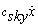) the semi-active damper will be in the “off” state. In the case that the semi-active damper will be capable of generating such a force, its continuously variable coefficient of damping csa will be defined from the equality of the sky-hook and semi-active force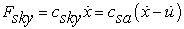(2)

and for the coefficient of damping the following equation holds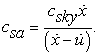(3)

The force in the semi-active damper is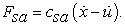(4)

This force can be realized in the same direction as the force in the sky-hook damper Fsky, only in the case that the direction of the relative velocity between the seat and the working machine floor and the absolute velocity of the seat are of the same direction. The condition can be written in the following form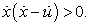(5)

In the case that this condition is met, the force in the semi-active damper is given according to equation (4). If this condition is not met, the semi-active damper will be in the “off” state. On the magnitude of the force Fsa will be applied an upper limit, so this damper would not generate higher forces than a real magnetorheological damper can do. In the “off” state of the damper a zero force Fsa will be considered, although in real (for example magnetorheological dampers) this force cannot be a zero force (moreover it is dependent on the relative velocity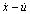). However, in practice this force practically does not affect the numerical results, if an appropriate magnetorheological damper is chosen. This condition is actually one of the criteria for the right choice of the magnetorheological damper. The advantage of the friction semi-active dampers is that they can easy generate the zero force in the “off” state, but in practice the magneto-rheological dampers are more used. These cannot give the zero force in the “off” state.

For the purpose of optimization we will consider the coefficient of the spring stiffness k and also the coefficient of damping of the sky-hook damper csky continuously variable in their search intervals, which will be shown in the following parts of the paper.

2.2. Dynamic and Mathematical Models of the Semi-active Suspension with the Dynamic Absorber

The dynamic model of the semi-active suspension with the dynamic vibration absorber is shown in the Figure 3. In this case there is an added mass m2 connected to the seat (under it) by a spring with stiffness coefficient k2 compared to the model without the dynamic vibration absorber shown in the Figure 1. In practice the realization of the dynamic vibration absorber is by an elastic beam, fixed to the seat frame, with a possibility to adjust the weight along it, which enables to adjust the parameter of the dynamic vibration absorber (its stiffness).

Mathematical model in this case can be written in the form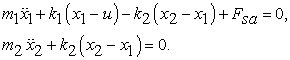(6)

For the semi-active damping force Fsa are valid the same conditions as were described above. The kinematic excitation will be considered with the same time response as it is shown in the Figure 2.

### 3. Suspension Parameters Optimization

A multi-objective function is considered in the form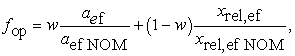(7)

where w is the weighting factor (gaining values from 0 to 1), aef is the effective acceleration of the seat described as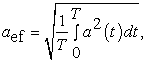(8)

where the acceleration of the seat a(t) is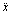in the case without the dynamic vibration absorber, the Figure 1, or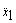in the case with the dynamic absorber, the Figure 3. The minimization of the effective acceleration aef leads to the maximization of the comfort of driver seated on the seat.

The second component of the objective function (7) defines the effective value of the relative displacement xrel = xu for the case without the dynamic absorber, the Figure 1, and xrel = x1u in the case with the dynamic vibration absorber, see the Figure 3. Similarly to equation (8) the following equation holds for the effective relative displacement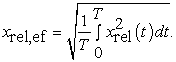(9)

T in the equations (8) and (9) represents the time of integration. The integration has to be sufficiently long in order to capture the dynamics of the system completely. Both the effective values aef and xrel,ef are in the equation (7) devided by their nominal values (defined for the mean values of the optimization variables csky and k in their search intervals, because the values of aef and xrel,ef are not commensurable. That would disable the numerical optimization.

3.1. Semi-active Suspension without the Dynamic Vibration Absorber

Because of the experimentally obtained kinematic excitation represented by displacement u(t) the optimiza-tion will be realized in the time domain. The Optimization Toolbox of Matlab  will be used. First it is necessary to transform the equation (1) into a system of the first order ordinary differential equations. If we use the transformation equations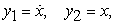(10)

then the system of the first order differential equations has the form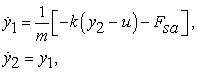(11)

where for force Fsa holds (after applying transformation equations (10) and their substitution to the equation (4))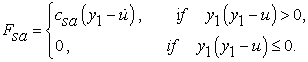(12)
3.2. Semi-active Suspension with the Dynamic Absorber

For the transformation of the mathematical model (6) into a system of the first order ordinary differential equations we will use transformation equations as follows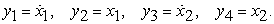(13)

We obtain a system of the four first order differential equations. Substituting equations (13) to equations (6) we aquire the following equations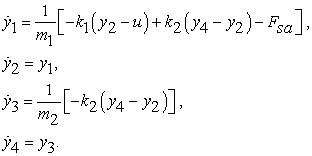(14)

### 4. Optimization Results

Two optimization variables are considered: the spring stiffness coefficient k and the sky-hook damping coefficient csky. Their search intervals are as follows: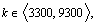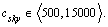Table 1 and Table 2 describe the optimization results for the case without the dynamic vibration absorber in dependence on the weighting factor w decreasing from value 1 to value 0.

From Table 2 flows that the values of the effective acceleration aef significantly change in dependence on the weighting coefficient w. On the other side the values of the effective relative displacement xrel,ef change only slightly. This leads to the conclusion that in this case the optimal solution is the one that completely prefers comfort (w = 1). The time responses of the relative displacement xrel(t) and accerelation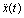of the seat are presented in the Figures 4 and 5.

#### Table 2. The effective acceleration and effective relative displacement for the case without the dynamic vibration absorber

Figure 5. Acceleration time response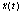for the case without the vibration absorber

In the case of the semi-active suspension with the dynamic vibration absorber was according to the optimization results from the case without the dynamic vibration absorber considered only the weighting coefficient w = 1. For the chosen value of the dynamic vibration absorber mass m2 = 6 kg by the 1-dimensional optimization the stiffness k2op = 1825.54 N/m of the dynamic vibration absorber spring was obtained. For the corresponding case without the dynamic vibration absorber (w = 1) the following optimum values were used: k1op = kop and csky,op. This allowed to determine the value of aef = 0.054978 m/s2 and xrel,ef = 0.001833 m. Comparing the values of the effective acceleration of the seat in both numerically simulated cases it is possible to allege that the decrease of the effective acceleration value of the seat in the case with the dynamic vibration absorber was about 9.6 %. The value of the effective relative displacement remained practically unchanged. The figure 6 shows the time responses of the seat displacement x1(t) for both cases (with and without the dynamic vibration absorber) and the Figure 7 shows the time responses of the seat acceleration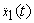for both cases.

### 5. Conclusion

In the paper two cases of the working machine seat semi-active suspension with and without the dynamic vibration absorber were modeled and optimized. It was shown that the use of the dynamic vibration absorber is for the considered kinematic excitation effective and leads to a decrease in the value of the seat effective acceleration. By multi-objective optimization a conclusion was obtained, that in the case of the semi-active suspension the optimal solution is the one that prefers comfort, because influence of the optimization weighting factor w on the effective value of relative displacement was not significant.

### Acknowledgements

This work was supported by grant project VEGA No. 1/1205/12.

### References

  He, Y., McPhee, J. “Multidisciplinary design optimization of mechatronic vehicles with active suspensions”, Journal of Sound and Vibration. 283 (1-2). 217-241. May 2005.In article CrossRef  Maciejewski, I., Meyer, L., Krzyzynski, T. “Modelling and multi-criteria optimisation of passive seat suspension vibro-isolating properties”, Journal of sound and Vibration, 324 (1-2). 520-538. July 2009.In article CrossRef  Segla, S., Reich, S. “Optimization and comparison of passive, active, and semi-active vehicle suspension systems”, in Proceedings of the 12th IFToMM World Congress on Mechanism Science, INRIA, Sophia-Antipolis, Besancon (France). 1221-1226. June 2007.In article  Georgiou, G., Verros, G., Natsiavas, S. “Multi-objective optimization of quarter-car models with a passive or semi-active suspension system”, Vehicle System Dynamics, 45 (1). 77-92. January 2007.In article CrossRef  Crews, J.H., Mattson, M.G., Buckner, G.D. “Multi-objective control optimization for semi-active vehicle suspensions,” Journal of sound and Vibration, 330 (4). 5502-5516. March 2011.In article CrossRef  Optimization Toolbox for use with MATLAB. Users guide, Version 2. The Mathworks Inc., Natick (USA, 2004.In article  Stein, J.G., Mucka, P., Gunston, T.P. “A study of locomotive dri-ver’s seat vertical suspension system with adjustable damper”, Vehicle System Dynamics, 47 (3). 363-386. May 2009.In article CrossRef  Hostens, I., Deprey, K., Ramon, H. “An improved design of air suspension for seats of mobile agricultural machines”, Journal of Sound and Vibration, 276 (1-2). 141-156. September 2004.In article CrossRef  Maciejewski, I. “Control system design of active suspensions”, Journal of Sound and Vibration, 331 (6). 1291-1309. May 2012.In article CrossRef  Ballo, I. “Comparison of the properties of active and semi-active suspension”, Vehicle System Dynamics, 47 (3). 363-386. May 2009.In article  Song, X., Ahmadian, M., Southward, S.C. “Modeling magnetorheological dampers with nonparametric approach”, Journal of Intelligent Material Systems and Structures, 16 (6). 421-432. May 2005.In article CrossRef  Ahmadian, M., Song, X., Southward, S.C. “No-jerk skyhook control methods for semiactive suspensions”, Journal of Vibration and Acoustics, 126 (4). 580-584. October 2004.In article CrossRef  Guglielmino, E. et al, Semi-active Suspension Control, Improved Vehicle Ride and Road Friendliness, Springer, Berlin, 2008.In article PubMed  Zilletti, M., Elliott, S.J., Rustighi, E. “Optimisation of dynamic vibration absorbers to minimise kinetic energy and maximise power dissipation”, Journal of sound and Vibration, 331 (7). 4093-4100. April 2012.In article CrossRef  Ji, J.C., Zhang, N. “Suppression of the primary resonance vibrations of a forced nonlinear system using a dynamic vibration absorber”, Journal of Sound and Vibration, 329 (10). 2044-2056. September 2010.In article CrossRef